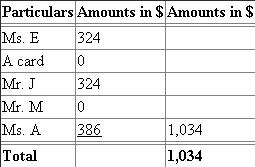# Quiz 7: Accounting for Sales and Accounts Receivable, and Cash Receipts

Recording sale made on account with a sales discount: A sale made on account would debit the account receivable as there is an increase in the asset with a corresponding credit to the sale account since revenue is credited. The following entries would be passed: Page 40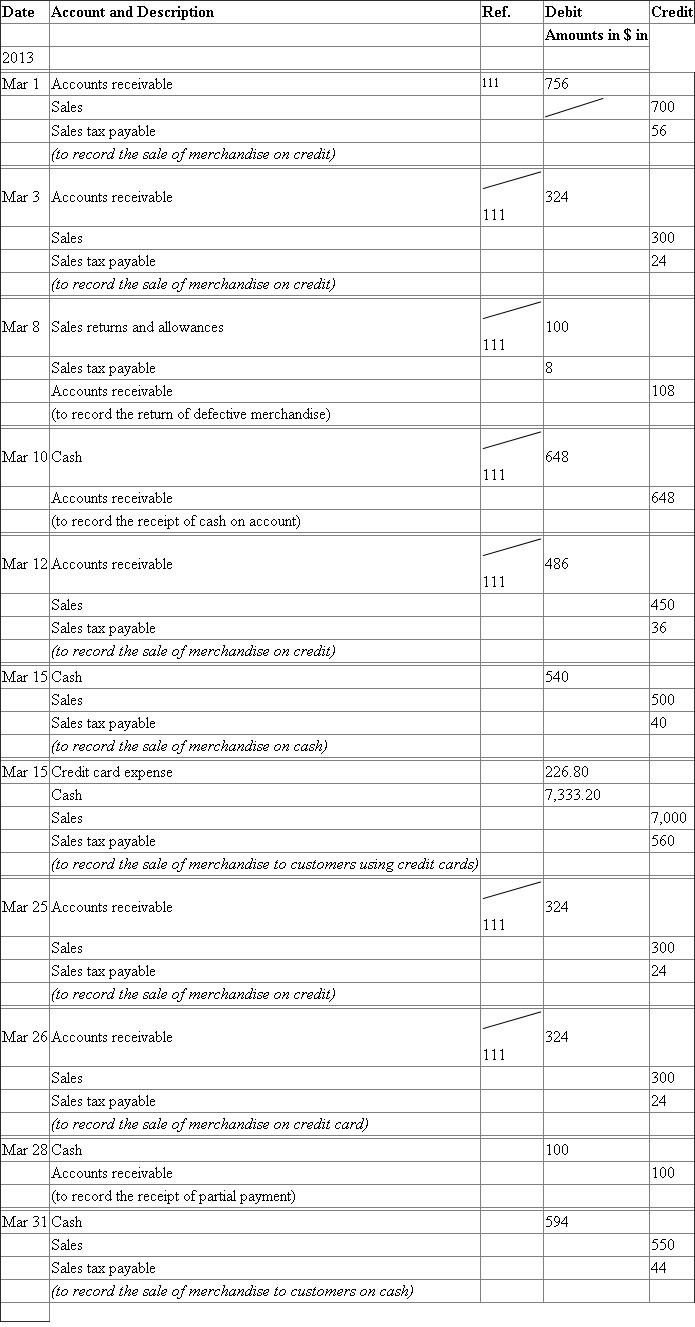Explanations: March 1: The amount in the accounts receivable account would be the total of \$700 and \$56. The amount of sales tax payable would be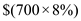March 3: The amount in the accounts receivable account would be the total of \$300 and \$24. The amount of sales tax payable would be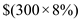March 10: The amount in the cash account would be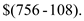March 12: The amount in the accounts receivable account would be the total of \$450 and \$36. The amount of sales tax payable would be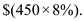March 15: The amount in the cash account would be the total of \$5,000 and \$400. The amount of sales tax payable would be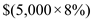March 15: The amount in the credit card expense account would be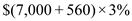The amount in the cash account would be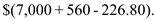The amount of sales tax payable would be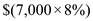March 25: The amount in the accounts receivable account would be the total of \$300 and \$24. The amount of sales tax payable would beMarch 26: The amount in the accounts receivable account would be the total of \$3,000 and \$240. The amount of sales tax payable would be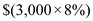March 31: The amount in the cash account would be the total of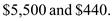The amount of sales tax payable would be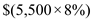March 31: The amount in the credit card expense account would be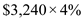The amount in the cash account would be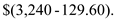The amount of sales tax payable would beMarch 31: The amount in the credit card expense account would be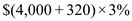The amount in the cash account would be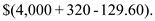The amount of sales tax payable would be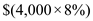The following is the general ledger of accounts receivable: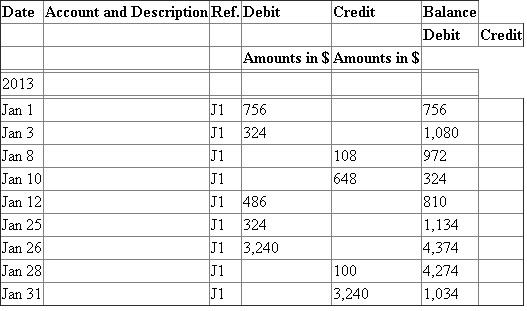The following is the subsidiary ledger of accounts receivable: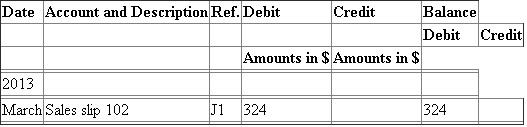The following is the general ledger of A: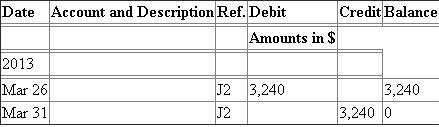The following is the subsidiary ledger of accounts receivable: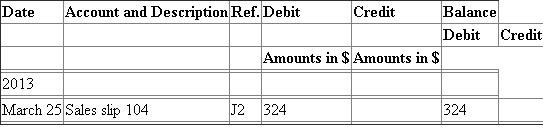The following is the general ledger of Mr. M: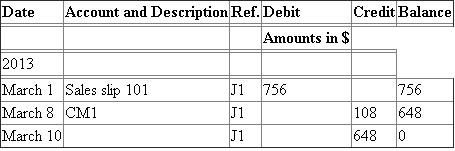The following is the general ledger of Ms. A: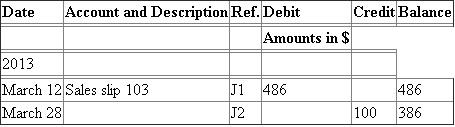The following is the schedule of the accounts receivable as on March 31, 2013: